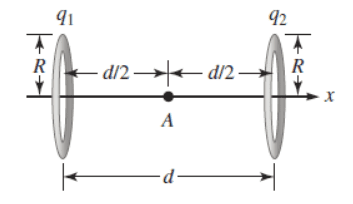# Expression for the magnitude of an electric field

• mousey
In summary, the problem involves calculating the electric field at point A due to two rings with different positive charges. The magnitude of the electric field is calculated using the line integral formula and the contributions from each ring are added to get the total electric field. The x-component of the electric field is enough to be calculated due to symmetry reasons, and the y and z components are found to be zero at point A. The integral for the upper half of the ring is calculated using the formula $$f(z)=\sqrt{R^2-z^2}$$ and for the lower half using $$\vec{r'}=-\sqrt{R^2-z^2}\hat y+z\hat z$$. Finally, the x-component is multipliedf

#### mousey

Homework Statement
Find an expression for the magnitude of the electric field at point A midway between the two rings of radius R shown in the figure below. The ring on the left has a uniform charge q1 and the ring on the right has a uniform charge q2. The rings are separated by distance d. Assume the positive x axis points to the right, through the center of the rings. (Use any variable or symbol stated above along with the following as necessary: k for Coulomb's constant. Assume q1 is greater than q2, and that both charges are positive.)
(picture in replies)
Relevant Equations
E= (kq)/d^2
k(q_1-q_2)/(R^2+x^2)^3/2You basically, have to consider one ring, let's say the left one, and calculate the magnitude of the electric field at A due to it, that is calculate the line integral $$\vec{E_1}=\int_{ring 1}\frac{k\rho_1 (\vec{r}-\vec{r'})dr'}{|\vec{r}-\vec{r'}|^3}$$, where $$\vec{r}=\frac{d}{2}\hat x$$,$$\rho_1=\frac{q_1}{2\pi R}$$, $$dr'=\sqrt{1+(f'(z))^2}dz$$ $$f(z)=\sqrt{R^2-z^2}$$, $$\vec{r'}=\sqrt{R^2-z^2}\hat y+z\hat z$$.

It is enough to calculate only the x-component of ##\vec{E_1}##, because the point A lies on the axis that passes through the center of the ring, hence due to symmetry reasons, the y and z components of ##\vec{E_1}## will be zero at point A.

Then calculate similarly the contribution ##\vec{E_2}## to the electric field from the ring to the right, and then take the sum $$\vec{E}=\vec{E_1}+\vec{E_2}$$ for the total electric field E at point A.

Last edited:
Thank you. I'm just wondering, the problem says q1 is greater than q2, and both are positive, so wouldn't I subtract E1-E2? Do I integrate both and then subtract?

Thank you. I'm just wondering, the problem says q1 is greater than q2, and both are positive, so wouldn't I subtract E1-E2? Do I integrate both and then subtract?
The fields add as vectors, snd as scalars along the x axis. But as scalars, one will have a negative value.
Yes, find each field separately before combining them.

Yes , well if you find that $$\vec{E_1}=A\hat x$$ where A is a scalar function of ##q_1,R,d## then you ll find that $$\vec{E_2}=-B \hat x$$ where B is another scalar function of ##q_2,R,d##, so that $$\vec{E_1}+\vec{E_2}=(A-B)\hat x$$

Just remember that when you do all the substitutions (for ##\vec{r},\vec{r'} , dr ## e.t.c) you ll end up with an integral that has ##\hat y## and ##\hat z## terms as well as the ##\hat x## term. Just ignore the first two terms (or maybe you can integrate as an excercise to prove that those integrals would be zero) and focus on the ##\hat x## term that is the x-component of the E-field.

And actually the way I 've setup ##\vec{r'}## is for the integral of the upper half of the ring. To calculate the integral for the lower half you got to set ##\vec{r'}=-\sqrt{R^2-z^2}\hat y+z\hat z##. This also means that when you calculate the x-component you got to multiply it by 2 (the x-component is the same for the upper half and for the lower half).

Last edited: